BackReturn Home

Eric Dollard's Foundations of Electrical Science

Eric Dollard is an electrical engineer. Long ago, he wrote many interesting articles for the Energetic Forum. Lamare, a user of that forum, was kind enough to make a backup of this information along with many useful resources. This page will be an attempt to distill some of that into a simplified form. It will assume a good understanding of arithmetic and a general familiarity with basic electricity as it is normally taught nowadays. [Reading Commonly Accepted Scientific Models Made Simple beforehand might help.]

Please note that none of this is necessarily endorsed by Eric. All of it will be colored by my personal interpretations. Extra explanatory notes are given in grey boxes and [square brackets]. Please feel free to skim over their contents if things do not make sense.

Contents

Primary Metrical Dimensions
Primary Physical Dimensions
The Four Primary Dimensions
Secondary Relationships
A Few Important Details
Quick Review Chart
Standard Physics Revisited (Interlude)
Transmission of Power
Parametric Variation
Colorado Springs & Wardenclyffe Tower
The Cosmic Induction Generator
The Multi-Wave Oscillator
The Integratron

Primary Metrical Dimensions

Time is symbolized by t and is measured in seconds
Space is symbolized by l and is measured in centimeters

Time is additive, linear:
Forward Time is t multiplied by +1
Reverse Time is t multiplied by -1

Space is multiplicative, exponential:

Counterspace Space
l-1 is span
space to the negative first power

Example: Along the side of the street, there is one utility pole every 150 feet. In other words, they have a span of one per 150 feet.
l+1 is distance
space to the positive first power

Example: It is a distance of 15 miles from here to the store.
l-2 is density
space to the negative second power

Example: The population density of coyotes on that land is one for every 50 acres.
l+2 is area
space to the positive second power

Example: There is a patch of land 1000 acres in size behind the store. In other words, the land has an area of 1000 acres.
l-3 is concentration
space to the negative third power

Example: The concentration of vitamin C in that orange juice is one daily requirement for every 8 oz. serving.
l+3 is volume
space to the positive third power

Example: The store only sells orange juice in containers with a half-gallon volume.

Important: Eric does NOT treat the terms "dimension" and "coordinate" as synonymous, as is often the case in modern mathematics. Time is a dimension, Space is a dimension, and we use how many ever coordinates that we need to describe a thing in relation to them.

In summary:

Aspects
of
The Dimension of Time
Aspects
of
The Dimension of Space
• Forward Time
• Reverse Time
• Space
• Counterspace

Important: There is a difference between the use of the word "Space" as a dimension, and "space" as an aspect of it (i.e.: l+1, l+2, and l+3).

Primary Physical Dimensions

Important: In order to use the conception of the Aether that lead to all of our modern electrical technology, Eric goes back to the pioneering work, mainly...

• The original concepts of Michael Faraday, the theoretical foundation of it given by James Clerk Maxwell, and Oliver Heaviside's application of that theory

• The experimental work of William Crookes and J.J. Thomson

• The creations of Nikola Tesla and Charles Proteus Steinmetz's mathematical analysis of them

Please keep this in mind as we continue...

There is a primordial substance or "Aether". To avoid all of the debates about its consitution, Eric treats it as simply a unique phase of matter:

1. Solid
2. Liquid
3. Gas
4. Plasma
5. Aether

 Modern physics also considers a fifth state of matter referred to as a "Bose-Einstein Condensate" (such as "superfluid Helium"). It is related. However, the general constitution and dynamics of the Aether were established by Faraday, Maxwell, and their contemporaries long before any of this terminology was used. For example, in an article from 1904 entitled "A Chemical Conception of the Aether", Dmitri Mendeleev treats it as a monoatomic or "inert" gas that comes before Hydrogen on the periodic table. These kinds of models of the Aether are very important, and should not be arbitrarily discarded. All of the above mentioned scientists proved their worth!

The concept of an Aether is fundamental to Electrical Science. As Eric puts it:
Electricity is embodied in the aetheric state of matter, or "proto-matter". Electricity is aether in a state of dynamic polarization; magnetism is aether in motion, dielectricity is aether under stress or strain.
In other words, there is One fundamental thing, which gives rise to two phenomena. This gives us our Primary Physical Dimensions:

Dielectricity is symbolized by the Greek letter Ψ ("psi") and is measured in Coulombs
Magnetism is symbolized by the Greek letter Φ ("phi") and is measured in Webers

Each of these has a distinct existence, fibrous structures that we will refer to as "tubes of force". However, they always appear together.

Dielectricity and Magnetism form an archetypal pair, a sort of Male and Female. Their union, or total Electrification, is symbolized by Q and is measured in Plancks.

We can represent this relationship by the equation:

Ψ × Φ = Q

We can think of crossing one tube of Dielectricity with one tube of Magnetism to form a Planck: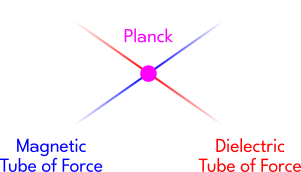A collection of both of these types of tubes is usually called an "electromagnetic field", or simply "electricity". Eric calls the crossing point of both tubes a "Planck", after the work of Max Planck. Max did some math that lead to the concept of a "photon". The "photon" is sometimes used in a manner analogous to Eric's Planck, but we will stick with his description instead. As he states, the Planck is simply "a quantum unit of electro-magnetic induction". A "quantum" is a discrete step, and Plancks can only change in quantities which are discrete steps (i.e.: they can only be represented by whole numbers, nothing fractional). The equation from which we derived the Planck is also similar to what is now known as a "Poynting vector", after the work of a scientist named John Henry Poynting. Eric generally eschews many of the modern physics interpretations of these older experiements and the theories that arose from them. This is because they have a tendency to obscure important information, especially within the practice of electrical engineering. For example... According to J.J. Thomson, the scientist who orginally discovered the "electron", an atom literally has thousands of "electrons" and each "electron" is the end of a single tube of Dielectricity. This is very different from the models of the atom that were formulated afterwards (such as the "Rutherford-Bohr Model"), which show atoms that contain a much smaller number of "electrons" (on the order of tens rather than thousands). Likewise, the "Electron Theory" states that the electromagnetic field is generated by the movement of "electron" particles within the atoms of a "conductor", such as a copper wire. These "electron" particles have mass. However, Eric is pointing out that these fields are generated as the result of something happening to an Aether and the phenomenon of electricity is "mass-free". In other words, while having a distinct existence that we can interact with, electricity is not "material" in the usual sense of the word. Not only does the "Electron Theory" affect the equations that are used to describe the situation (by introducing concepts like mass), it also leads to interpretations of how various electrical components function that can be misleading. For example, "capacitors" are devices which store Dielectricity. They are essentially a piece of glass (an "insulator") sandwiched between two metal plates. In "Electron Theory", capacitors are treated as if "electrons" accumulate on the surface of these plates. But we can use a "dissectible capacitor" to show that Dielectricity is actually held within the glass itself! In other words, it goes towards Counterspace. The "insulator" is really an "absorber". Similarly, Magnetism is completely expelled from a "conductor" during the phenomenon of "superconductivity". This is called "Meissner Effect". This means that "conductors" could be more properly thought of as "reflectors". Notice that we regularly use the terms "conductor" and "insulator" in ways that are opposite from what they are actually doing! There are quite a few things that seem backwards within modern physics.

The Four Primary Dimensions

We now have all four Primary Dimensions:

Metrical
Time (t in seconds)
Space (l in centimeters)

Physical
Dielectricity (Ψ in Coulombs)
Magnetism (Φ in Webers)

Every other value that we can get will be built up from these four!

 Note the similarity of this to works like The Reciprocal System of Theory and Symmetric Field Physics. Eric's math is incredibly useful for giving a tangible, engineering basis to many alternative models. It is absolutely astounding how far one can take it because everything is "electrical" in nature. Hopefully, some of this will become more clear as we continue.

Secondary Relationships

All electrical components and devices are simply different ways of interacting with the Aether through the tubes of force that they form. We can map out these relationships mathematically...

We already saw how to get Electrification (Q in Plancks):

Ψ × Φ = Q

If we change the amount of Dielectricity or Magnetism that there is within a given space over time, then we get two new values:

• Displacement Current is symbolized by I and is measured in Amperes
Electro-Motive Force (or EMF) is symbolized by E and is measured in Volts

To put these relationships another way: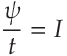and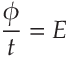Eric refers to the first equation as "Maxwell's Law of Dielectric Induction". It represents how Displacement Current flows through a dielectric (i.e.: an "insulator").

Eric refers to the second equation as "Faraday's Law of Magnetic Induction". It represents the build up of EMF around a metallic structure (i.e.: a "conductor").

If we take both amounts (i.e.: Dielectricity and Magnetism simultaneously), then we get this relationship: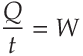• Energy is symbolized by W and measured in Joules

Like the word "Aether", the term "Energy" can be used in a variety of different ways, but this is how one would define it mathematcially within the context of electrical engineering! In physics generally, "Energy" is the "ability to do work" (i.e.: the ability to move something).

To recap, we now have I, E, and W. We can get more measures by rearranging these terms...

Dividing E by I gives us: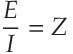• Impedance is symbolized by Z and is measured in Ohms

This would seem to indicate that Impedance is the build up of EMF being dampened out by the flow of Displacement Current.

Inversely, dividing I by E gives us: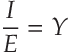• Admittance is symbolized by Y and is measured in Siemens

This describes a situation opposite to the previous. Admittance is the flow of Displacement Current being dampened out by the build up of EMF. In this case, one is the denial of the other.

What if we multiply instead? Then, we get:

E × I = P

• Electrical Activity (also known as "Power") is symbolized by P and measured in Watts

While they are related, "Power" is often confused with "Energy". In physics generally, "Power" is defined as the rate at which work is done. In other words, "Energy" is how much is moved, and "Power" is how fast that happens.

Remember, each term can still be represented by the equation which formed it, so we can also represent the previous equation as: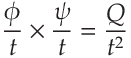Can you see how the two previous equations are the same thing written in different ways? We will be doing a lot of this type of equation rearrangement as we continue. Don't worry! If we need more sophisticated math, we will explain it as we go along.

A Few Important Details

Why are we bothering with all of this? Why not just use conventional, modern-day electrical theory? Because this approach makes clear some very subtle, but very important, relationships that are a bit tricky to get ahold of otherwise. For example...

There are actually two different situations that use Amperes as a unit of measurement:

• Displacement Current, represented here by an uppercase I
Magneto-Motive Force (MMF), represented here by a lowercase i

 This latter one is related to what most people would call "Current" now (or more properly, "Conduction Current"). If EMF describes the build up of the Magnetic tubes of force around a metallic structure, then MMF would describe the flow of Magnetic tubes of force through it.

There are also two different situations that use Volts as a unit of measurement:

Electro-Motive Force (EMF), represented here by an uppercase E
• Electrostatic Potential, represented here by a lowercase e

 This situation is inverted from the previous. If Displacement Current describes flow of the Dielectric tubes of force through a dielectric, then Electrostatic Potential would describe the build up of Dielectric tubes of force around it.

Notice that the Displacement Current I and Electrostatic Potential e are related to the movement and build up of Dielectricity, while Magneto-Motive Force i and Electro-Motive Force E are related to the movement and build up of Magnetism.

 The former (I and e) are related to what are now called "Electric Circuits", while the latter (i and E) are related to what are now called "Magnetic Circuits". In a more general physics sense, we can think of that process of build up as a change in "Potential Energy" and the movement as a change in "Kinetic Energy".

Similar to how we used I and E to form more relationships, we can use i and e to do the same.

Let's take Ψ and divide it by e: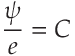• Capacitance is symbolized by C and is measured in Farads

Capacitance is the ability for something to store Dielectricity [until it reaches "Dielectric Breakdown"]. The less Capacitance that there is, the more Electrostatic Potential. Eric calls this "The Law of Dielectric Proportion".

Since division is the "inverse operation" of multiplication, we can also re-write this equation as:

e × C = Ψ

Now, let's take Φ and divide it by i: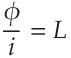• Inductance is symbolized by L and is measured in Henrys

Inductance is the ability for something to store Magnetism [until it reaches "Saturation"]. The less Inductance that there is, the more MMF. Eric calls this "The Law of Magnetic Proportion".

Again, since division is the inverse operation of multiplication, we can re-write this equation as:

i × L = Φ

We can also describe the rate at which this storage happens by taking the ratio of C or L to t. In other words, how does Capacitance or Inductance change over time?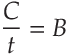• Susceptance is symbolized by B and is measured in Siemens

Notice that Admittance Y was also measured in Siemens.

Next, let's take L: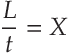• Reactance is symbolized by X and is measured in Ohms

Notice that Impedance Z was also measured in Ohms.

Both Susceptance and Reactance represent the storage of Energy within a system changing. While it might seem a little strange at first, we use the exact same equations to describe Energy dissipating from a system. This is because, whether it is entering or leaving the system, it is still a change in time. To distinguish the two situations from one another, we refer to them by different names.

The loss of Dielectricity to the dielectric is represented by the equation: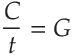• Conductance is symbolized by G and is measured in Siemens

Again, we have something measured in Siemens, like Admittance Y and Susceptance B.

The loss of Magnetism to the metallic structure is represented by the equation: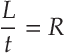• Resistance is symbolized by R and is measured in Ohms

Yet again, we have something measured in Ohms, like Impedance Z and Reactance X.

Both Conductance and Resistance appear as heat within the dielectric and the metallic structure, respectively. In the latter situation, the "electron" represents the conversion of Energy into noise. The "electron" does not convey electricity within this model. The tubes of force do. In turn, the tubes of force come from the Aether.

Quick Review Chart

The use of all of these units might seem complex, so let's quickly review the equations of the previous sections:

Symbol Name of Phenomenon Unit of Measure Representative Equation
Ψ Dielectricity Coulombs
Φ Magnetism Webers
Q Electrification Plancks Ψ × Φ = Q
I Displacement Current AmperesE Electro-Motive Force (EMF) VoltsW Energy JoulesZ Impedance OhmsY Admittance SiemensP Power Watts E × I = P
i Magneto-Motive Force (MMF) Amperes
e Electrostatic Potential Volts
C Capacitance FaradsL Inductance HenrysB Susceptance SiemensX Reactance OhmsG Conductance SiemensR Resistance OhmsThese distinctions are going to become quite valuable to us as we try to explain more obscure phenomena.

Standard Physics Revisited (Interlude)

 These types of relationships are usually introduced in a simplified way that is referred to as "The Ohm's Law Triangle". It looks something like this: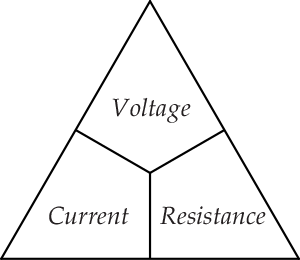In this context, "Current" means MMF (what we symbolized by a lowercase i above) and "Voltage" means EMF (what we symbolized by an uppercase E above). "Resistance" has the same meaning (what we symbolized by R above). The reason why they are arranged in this manner is because it makes it easy to remember how they interrelate mathematically: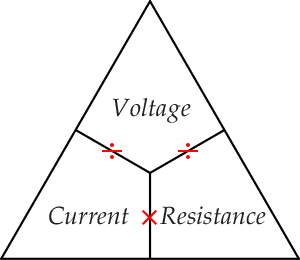And from this, we can derive the following equations: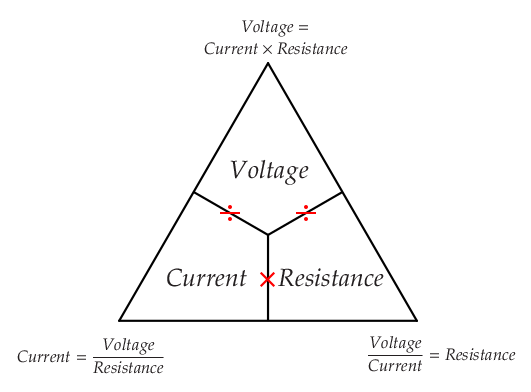Similar things are sometimes done within physics for the equations related to Space and Time: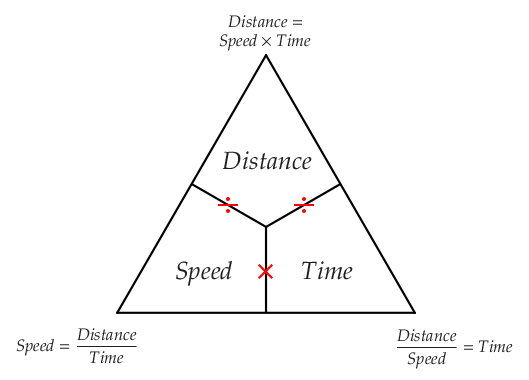...and for those related to density: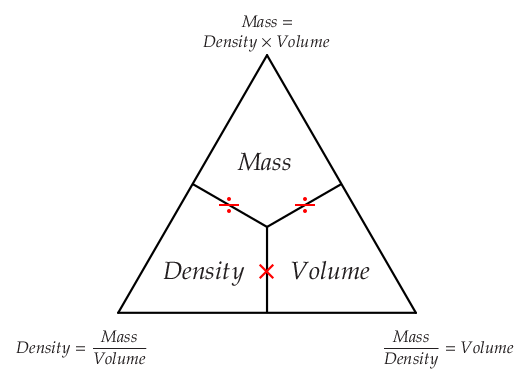"Mass" is an amount of matter and "volume" is an amount of Space. "Density" is usually defined as the amount of mass within a given volume. While these relationships can be useful in some situations, they can also be extremely misleading if one is not careful. We will share a few things that we think are helpful to keep in mind: 1. Notice that "The Ohm's Law Triangle" refers only to Magnetism (i and E) and says nothing about Dielectricity (I and e). Even when expanded into the larger "Ohm's Law Wheel", many electrical phenomena are completely absent. In general, there seems to be a gap in electrical education when it comes to the understanding of Dielectricity (or what is more commonly referred to as the "Electric Field", not to be confused with the "Electromagnetic Field"). This is compounded by the fact that some treat the science of "Electrostatics" as a historical oddity with little practical value. 2. Traditional physics focuses a lot on Space and Forward Time, whereas the concepts of Counterspace and Reverse Time might seem almost non-existent. However, "relatively unknown" does not necessarily mean "useless". For example, Counterspace is related to the branch of mathematics known as "Projective Geometry". It is useful for describing electrical phenomena as they appear within Nature. A few researchers who have explored this type of math are George Adams, Olive Whicher, Lawrence Edwards, and Nick Thomas. They have given a solid basis to the study of what Rudolf Steiner calls the "Etheric Formative Forces". Some have approached it in an intuitive, qualitative, and psychological way too (e.g.: Guenther Wachsmuth, Ernst Lehrs, et al.). The term "Ether" is often synonymous with what we have been referring to here as "Aether". One can find a lot of work related to this subject, but it is sometimes disregarded by being labelled as "dated", "fringe", or disrespectfully, as "woo-woo". Not all of it may be of equal use in engineering, but one's patience and kindness are needed. Truth can appear anywhere and at anytime. It cannot be emphasized enough that, while humanity has definitely gained plenty of useful knowledge in the development of science, certain understandings have also become lost. And the only way to retrieve them is to be willing to carefully review what we may think we already know. If we trace out the history of the Aether concept, we can see why many physicists chose to discard it despite its importance within the development of electrical technology. However, what is essentially the same concept often goes by different names (e.g.: "vacuum", "spacetime", etc.), and has a tendency to be approached mathematically instead of experimentally (i.e.: "theoretical physics"). 3. Analogies can help make something more clear by giving a picture to aid in visualization, or by connecting reasoning to something familiar. Yet, at the same time, they can hide important information when taken a little too literally. For example, "Electron Theory" is partially founded upon the analogy that can be made between "The Ohm's Law Triangle" and "The Density Triangle" given above. Physicists adopted these methods because they once measured the intensity of electrical effects by how strongly they pushed upon scales, as if electricity was a substance that could be weighed [*see "electromechanical" meters like the "Ampere Balance"]. How can something affect matter without itself being "material"? Electricity, more than any other Natural phenomenon that humans have learned to work with, embodies a sense of the mysterious. It can gently pass like a ghost through solid objects or utterly obliterate them in a violent explosion. Early electrical scientists, such as Lord Kelvin, described atoms as akin to "vortices" of Aether. J.J. Thompson, and several others, developed models of "vortex atoms" throughout this period of history (around the late 1800's). And many other less well-known researchers, like Carl Frederick Krafft, continued to develop the idea of atoms being vortices of Aether long after that time.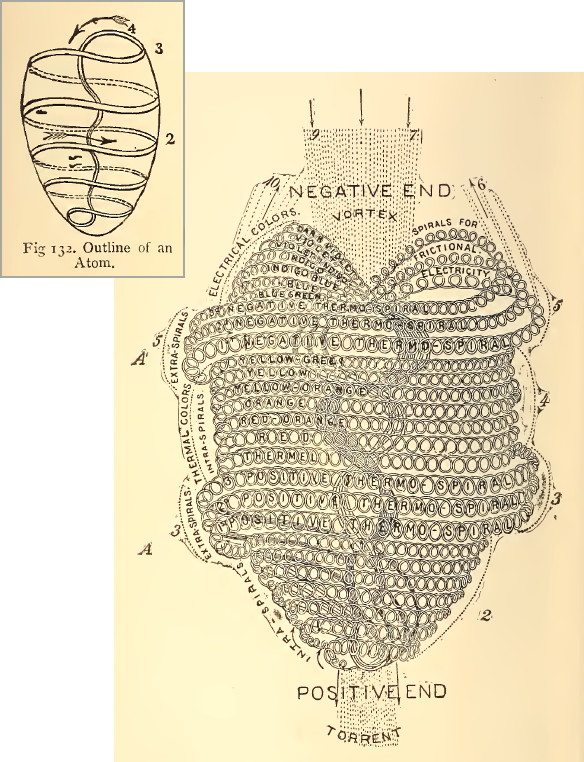Diagrams from pgs. 97 and 102 of the book The Principles of Light and Color by Edwin Babbitt (circa 1896) Up unto this day, there are several different models of the atom used interchangably within chemistry to describe various phenomena (e.g.: "electron orbitals" are used for describing the geometry of how atoms bond together and "electron shells" are used for describing the properties of different materials, such as whether it would make a good "conductor" or "insulator"). Generally, there seems to be a lot of confusion as to the "electronic structure" of the atom, and by extension, the role that various "sub-atomic particles" play within it. Just as there are many different models of atom, there are many different models for "sub-atomic particles". These are not "new" concepts either. For example, in 1915, the chemist/physicist Alfred Parson developed a model of the "electron" that treated it as a bundle of "electrical fibers" in a ring shape. Notice how different this is from the J.J. Thomson idea of an "electron" that we mentioned earlier (i.e.: the end of a Dielectric tube of force). Similar to the image of the vortex atom depicted above, we might think of it as a braiding together of tubes of force. It is like an "electric field" folded in on itself, instead of appearing around or within an electrical device. This makes clear some of the more idiosyncratic ideas about "electrons" that are found within the context of modern "quantum physics". We will quote an interesting interview with the electronics inventor Carver Mead as another example: So early on you knew that electrons were real. The electrons were real, the voltages were real, the phase of the sine-wave was real, the current was real. These were real things. They were just as real as the water going down through the pipes. You listen to the technology, and you know that these things are totally real, and totally intuitive. But they're also waves, right? Then what are they waving in? It's interesting, isn't it? That has hung people up ever since the time of Clerk Maxwell, and it's the missing piece of intuition that we need to develop in young people. The electron isn't the disturbance of something else. It is its own thing. The electron is the thing that's wiggling, and the wave is the electron. It is its own medium. You don't need something for it to be in, because if you did it would be buffeted about and all messed up. So the only pure way to have a wave is for it to be its own medium. The electron isn't something that has a fixed physical shape. Waves propagate outwards, and they can be large or small. That's what waves do. So how big is an electron? It expands to fit the container it's in. That may be a positive charge that's attracting it--a hydrogen atom--or the walls of a conductor. A piece of wire is a container for electrons. They simply fill out the piece of wire. That's what all waves do. If you try to gather them into a smaller space, the energy level goes up. That's what these Copenhagen guys call the Heisenberg uncertainty principle. But there's nothing uncertain about it. It's just a property of waves. Confine them, and you have more wavelengths in a given space, and that means a higher frequency and higher energy. But a quantum wave also tends to go to the state of lowest energy, so it will expand as long as you let it. You can make an electron that's ten feet across, there's no problem with that. It's its own medium, right? And it gets to be less and less dense as you let it expand. People regularly do experiments with neutrons that are a foot across. A ten-foot electron! Amazing. It could be a mile. The electrons in my superconducting magnet are that long. It is okay if this quote is not entirely clear. We just want to highlight the fact that the ideas of "electrons" that have been developed for use within chemistry and electronics are different from the ideas used by those who developed the foundations of electrical engineering. And further, because of those changes, layers of complexities have accumlated upon the situation that can make it hard to understand and duplicate that work.

Transmission of Power

Parametric Variation

Colorado Springs & Wardenclyffe Tower

The Cosmic Induction Generator

The Multi-Wave Oscillator

The Integratron

[In Progress...]